## Math Fact Benchmarks: Why Writing Speed Matters

Math drills are used to help students learn their basic single-digit math facts. The goal is to help students answer basic math facts from recall and develop automaticity. The only way to tell if a student is recalling the answer rather than figuring it out is by using timed math drills as benchmarks. But students cannot learn how to recall a full page of math facts by drilling on them. The drills will likely cause anxiety and frustration for students. Here are some common pitfalls that are wise to avoid when teaching students basic single-digit facts.

## Common Problems With Math Benchmarks

Schools typically set “benchmarks” to evaluate whether students have achieved their educational goals.  When it comes to math fact memorization, schools will set benchmarks to distinguish between knowing the answers by a quick recall from the slow process of figuring out the facts. There are two main problems with these benchmarks.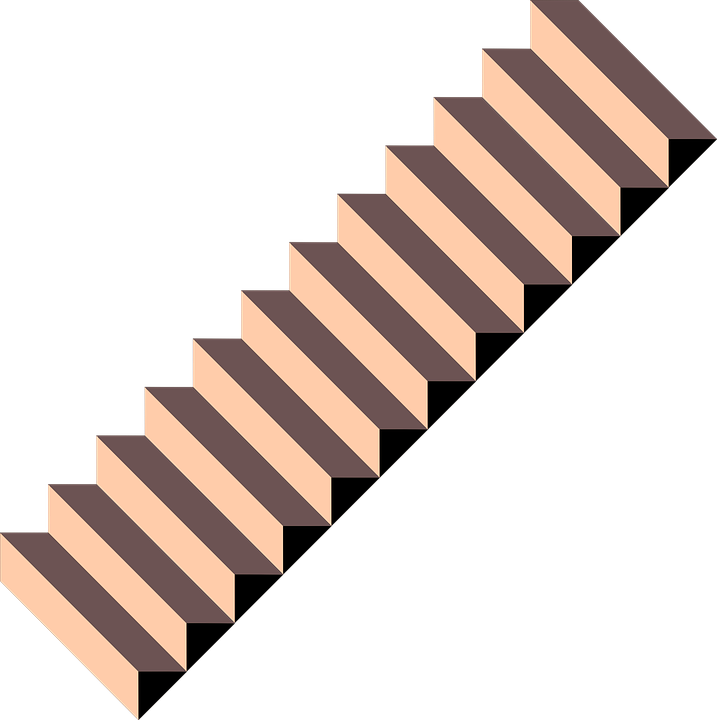### Learned one at a time.

The first problem is that math facts are learned individually and students meet the benchmark one problem at a time. The benchmark should be to answer a problem in less than a second. If they can, then it would be clear which math facts a student has memorized and which ones they don’t. It is more common for schools to give tests on all the facts in an operation and these tests can’t tell the schools which facts are memorized. A more meaningful report would be which facts in an operation can a student answer instantly.### Time to write the answer.

The second main problem is that no matter how well students know facts, they cannot write answers to facts any faster than they can write. Elementary students vary in speed and can write answers anywhere from 10 answers in a minute to 50 in a minute. If a student can answer 40 problems in a minute, they have achieved mastery of math facts. But if they can’t write answers that quickly, they can not meet their school’s benchmark. In order to set a reasonable standard, teachers need to know how fast students can write and a writing speed test will help determine this.

## Common Core Math Fact Fluency Need Not Cause Anguish

The Common Core Idea says that students must have a quick recall of math facts to progress successfully in math. For some reason, almost all children have memorized 2+2=4 and can answer this problem from recall. It is not stressful or hard and will not cause math phobia. So, we know that learning how to answer a math problem instantly from recall is doable and can be done without being stressful. The challenge is that there are a lot of facts to learn, but taken a few at a time, they can all be learned equally easily.

To do this though, it takes a systematic effort, daily practice, and careful monitoring. Teachers need a facts program of some kind to help their students commit a large number of facts to memory. Before developing the design principles behind Rocket Math, I had thought this was a nearly impossible task. But in the last 20 years, I have seen how Rocket Math has been used successfully to teach math facts to students. With plenty of time, patience, and encouragement, students can learn to recall math facts from memory and with Rocket Math they can do this while having fun.

## Rocket Math Writing Speed Test

Here is a simple easy-to-use Writing Speed Test to help figure out how fast a student is able to answer when they have all the facts in an operation memorized. The test is a mix of one and two-digit numbers so it works with addition and multiplication.

Simply give the test to your students and have them write the numbers they see in each box for one minute. You will be able to find out how many boxes they can complete in one minute. That number is the upper limit of math fact problems you can expect them to be able to answer–if they are answering from recall rather than by figuring them out.

### Writing speed is number of boxes completed.

When the student has finished the test, you will have the number of boxes they can complete in a minute. You can expect the student to be able to finish at least 80% of that number if they can recall the facts instantly.  If the student can answer 90%, they don’t need any fact work. If they are between 80 and 90% of that number, they are good, but more facts work would help them. Anything below 80% and they are having to stop to figure out some of the facts and need more work.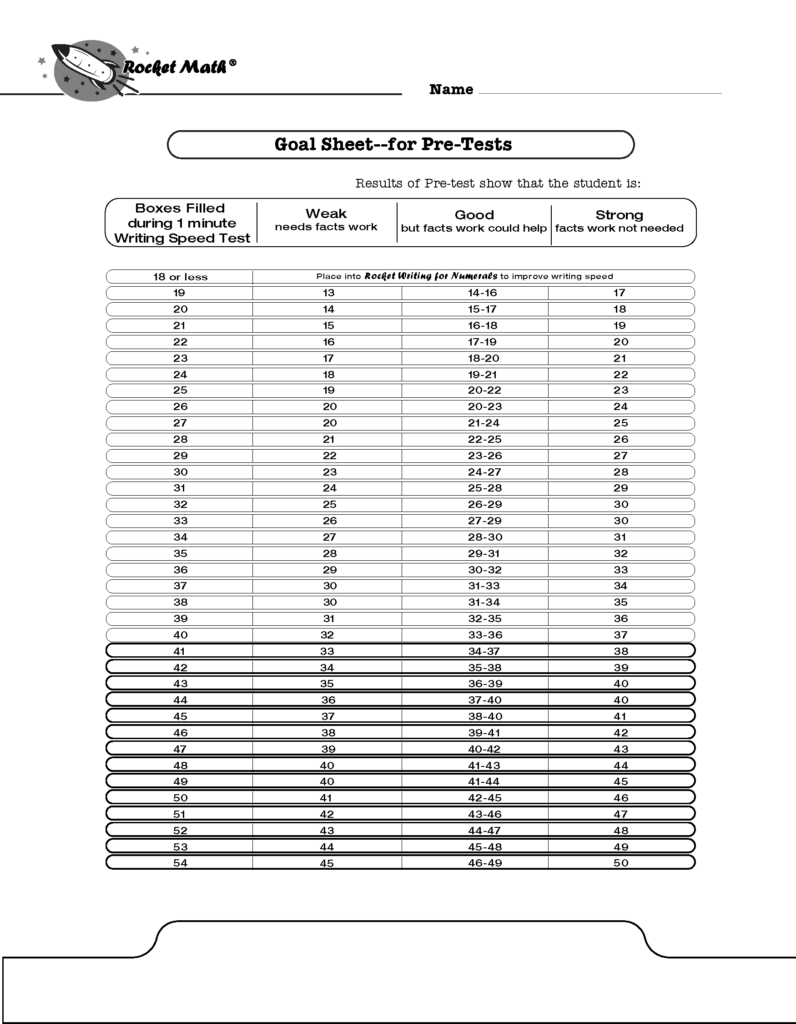If you’re not interested in doing the math over and over, here is a goal sheet for pre-tests, where the numbers are worked out for you. You can print it from this link.

### Benchmarks must be based on writing speed.

Benchmarks that don’t take into account the speed at which children can write, leave a lot of children with an impossible expectation. Asking children to do something they are physically incapable of doing will cause a lot of unnecessary anguish.

## Kindergarten Math BenchmarksStudents should be taught how to write numerals correctly and efficiently in kindergarten. The methods of drawing numerals that children invent on their own can be slow, cumbersome, and inefficient – causing them to have a slow writing speed later. Rocket Math Writing for Numerals is a systematic method that will help students learn how to form their numerals. Benchmarks for kindergarteners should focus on the speed of writing numerals, which takes a good amount of practice and instruction.

Kindergarten Numeral Writing Fluency Benchmarks (digits)

• Mid-year
• 20 digits per minute
• End of year
• 40 digits /minute

## First-grade Math BenchmarksFirst-grade students need to be fluent in writing numerals. Not every kindergarten does this, so teachers should test and give some kind of numeral writing program to students who do not meet these writing standards.

First Grade Numeral Writing Fluency Benchmarks (digits)

• Start of year
• 40 digits per minute
•  Mid-year
• 60 digits per minute
•  End of year
• 60 digits per minute

We also want first-graders to learn addition facts to the level of instant recall. The student’s writing speed is the number of boxes they can complete in one minute.

First Grade Math Fact Fluency Benchmarks (problems)

• Start of year
• Addition 20% of writing speed
•  Mid-year
• Addition 40% of writing speed
• End of year
• Addition 80% of writing speed
• Subtraction 20% of writing speed

## Second-grade Math Benchmarks

In second-grade we want students to master subtraction facts as well as addition.

• Start of year
• Addition 80% of writing speed
• Mid-year
• Addition 80% of writing speed
• Subtraction 40% of writing speed
• End of Year
• Addition 80% of writing speed
• Subtraction 80% of writing speed

## Third-grade Math BenchmarksIn third-grade it is important that students master subtraction and begin working on multiplication.

• Start of year
• Addition 80% of writing speed
• Subtraction 80% of writing speed
• Mid-year
• Addition 80% of writing speed
• Subtraction 80% of writing speed
• Multiplication 40% of writing speed
• End of year
• Addition 80% of writing speed
• Subtraction 80% of writing speed
• Multiplication 80% of writing speed

## Fourth-grade Math Benchmarks

In fourth-grade it is important for the students to have multiplication mastered and begin division.

• Start of the Year
• Addition 80% of writing speed
• Subtraction 80% of writing speed
• Multiplication 80% of writing speed
• Mid-year
• Addition 80% of writing speed
• Subtraction 80% of writing speed
• Multiplication 80% of writing speed
• Division 40% of writing speed
• End of year
• Addition 80% of writing speed
• Subtraction 80% of writing speed
• Multiplication 80% of writing speed
• Division 80% of writing speed

## Fifth-grade (and up) math fact benchmarksIn fifth-grade it is important for the students to have mastered their basic math facts in addition, subtraction, multiplication, and division.

• Start of year
• Addition 80% of writing speed
• Subtraction 80% of writing speed
• Multiplication 80% of writing speed
• Division 80% of writing speed
•
•
• Mid-year
•  Addition 80% of writing speed
• Subtraction 80% of writing speed
• Multiplication 80% of writing speed
• Division 80% of writing speed
• End of year
• Addition 80% of writing speed
• Subtraction 80% of writing speed
• Multiplication 80% of writing speed
• Division 80% of writing speed

All the 1-minute pre-tests and the writing speed tests and the goal sheet can be found on this page https://rocketmath.com/pre-tests/.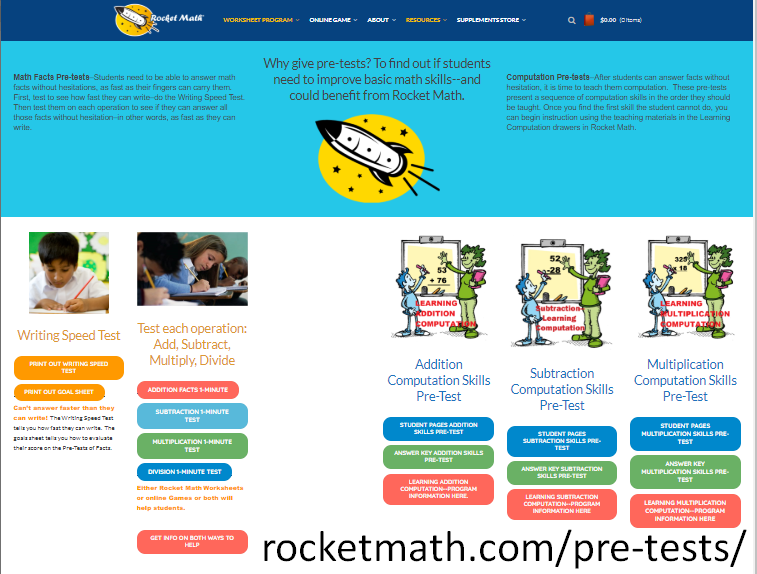## Rocket Math Is A Fun Way For Students To Learn

Rocket Math has a systematic approach to teaching students how to become fluent in basic math facts and still have fun learning math. Math can seem daunting to learn and if not taught properly, using a good math program, students can build a math phobia. Teachers have been using Rocket Math for over 20 years now and believe it to be an invaluable tool to help their students learn math.

## Math Facts Practice Online: Add, Subtract, Multiply, Divide

Searching for a program to practice math facts online that will help your learner power through their math facts and have fun?

Rocket Math Online Game offers effective addition, subtraction, multiplication, and division practice, and students have a blast doing it! Why do they love the game? The game helps students quickly and methodically memorize math facts, which means less frustration and more fun! The game also shows students their progress with exciting, rocket ship-themed graphics and audio to keep them motivated to learn more. It turns out that students learn better and are more motivated to continue when they can clearly see their progress. Who knew?

## How Rocket Math’s online math game worksToo many children still count on their fingers to figure out basic addition facts. If a child continues to spend hours counting on their fingers, it is a sure way to make them hate math. Professors of education frequently teach that “all rote learning is bad for children.” This is not true when it comes to math facts. Memorizing basic facts is a necessary step (to free up working memory) on the path to higher-order thinking in math, and by skipping math fact memorization, teachers are handicapping their students. As a result of the dogma against memorization, few new teachers have any idea how to effectively help their students memorize.

For example, giving students a worksheet full of problems that they haven’t been able to memorize isn’t going to help. Nor will a computer practice game that randomly gives problems for students to solve. A good math program will provide students with a few math facts at a time to work through before adding more.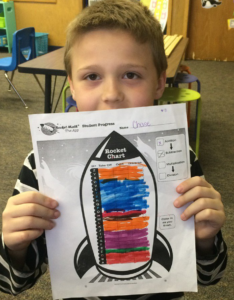Rocket Math Online Game does just this. Starting with two math facts and their reverse, the game won’t let the student move on until they can answer these math problems instantly. Rocket Math Online Game will then gradually, carefully, and systematically add new facts to those already learned. Students have to answer in 3 seconds or less, or they have to do that part over until they can answer the math fact immediately. They will work through set A to set Z with 26 levels in three phases; Take-Off, Orbit, and Universe. Each time they complete a set, the tile for that set explodes and falls away. As students progress through the levels, they can fill out the Rocket Chart to see their progress and stay motivated.

## Addition Math Facts Practice in Rocket Math Online Game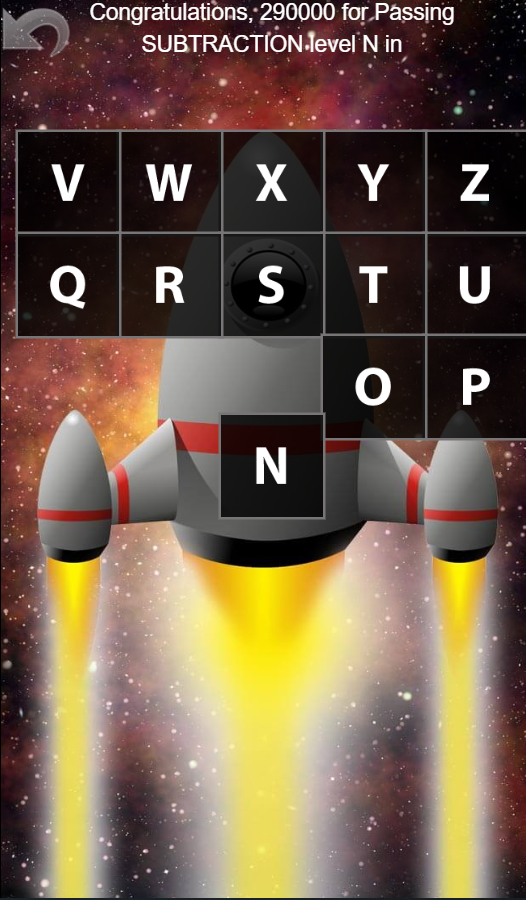First-grade students should begin working on the first Learning Track: Addition 1s through 9s math facts and have all those facts memorized first. With Rocket Math Online Game, there are three Learning Tracks to choose from for your first-grade class.

• The Basic Learning Track
• 1. Addition 1s through 9s
• The Alternative Learning Track: learning addition and subtraction facts in families
• 5. Fact Families (+, -) to 10
• Optional Learning Track
• 7. Add to 20

If you notice a student is taking more than a week to pass a level in sets A-Z of Addition 1s through 9s, that’s a sign for you to intervene. Often this means that the child is struggling and needs to practice more. They need to logon and practice at home in addition to their practice in school. The first graders who can finish the Learning Track for Addition 1s though 9s, can move on to the Optional Learning Track, Add to 20. Advanced first graders who are very quickly mastering facts can certainly move into the Learning Tracks recommended below for 2nd grade.

There is an alternative sequence of learning addition and subtraction facts, through Fact Families.  Fact Families introduces addition and subtraction facts at the same time in “families” such as 1+3, 3+1, 4-3, 4-1. Because the facts are introduced in families students are able to switch back and forth between addition and subtraction as they are learning. Rocket Math breaks up the fact families into a Learning Track with addition and subtraction facts up to 10 to begin in first grade and then a second Learning Track of facts from 11 that follows after, either in first grade or second.

## Subtraction Math Facts Practice in Rocket Math Online Game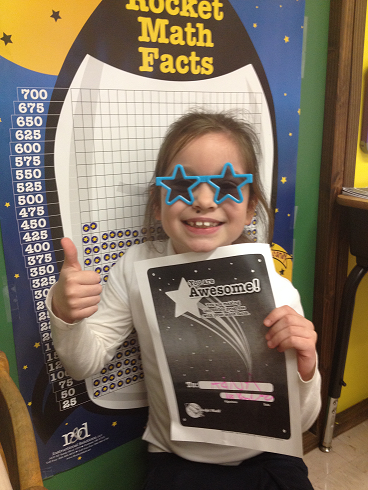Many teachers think subtraction facts are harder for children to learn.The reason they seem harder to learn is that most children don’t fully master addition before they start memorizing subtraction facts. When that happens, the two operations interfere with one another (officially, it’s known as proactive and retroactive inhibition), and subtraction facts become harder to learn.

Students who work through the addition sets in Rocket Math Online Game, will not find this to be a problem. Once the student has mastered the addition facts, they will quickly recognize that subtraction facts are “the opposite” of addition. The interference does not happen, and the students will feel good about their progress and learn to do computation with ease.

Rocket Math Online Game offers these Learning Tracks for second graders to master subtraction:

• The Basic Learning Tracks
• 1. Addition 1s through 9s
• 2. Subtraction 1s through 9s
• The Alternative Learning Tracks: learning addition and subtraction facts in families
• 5. Fact Families (+, -) to 10
• 6. Fact Families (+, -) from 11
• Optional Learning Tracks
• 7. Add to 20
• 8. Subtract from 20

Second graders who did not learn addition Math Facts in first grade must focus on addition facts first. After they have gotten through Set Z of addition, they can move on to 2. Subtraction 1s through 9s.

Second-grade students who complete addition and subtraction 1s-9s can start Add to 20 and then go on to Subtracting from 20.

As noted above,  learning by fact families is an alternative route to learning basic addition and subtraction facts.  The first Learning Track would be #5 Fact Families (+, -) to 10 followed by #6 Fact Families (+, -) from 11.

## Multiplication Math Facts Practice in Rocket Math Online Game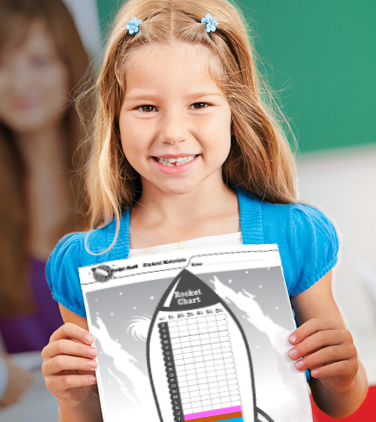Being able to multiply is harder than addition or subtraction because you can’t count on your fingers. While it is necessary for students to memorize the “times facts,” they are seldom systematically taught. Preservice teachers are frequently taught that “rote learning is bad for children.” This is not true, but as a result, most new teachers have no idea how to effectively help their students memorize. Memorizing basic facts is a necessary step (to free up working memory) on the path to higher-order thinking in math, and by skipping math fact memorization, teachers are handicapping their students.

Rocket Math Online Game offers these Learning Tracks for third graders to master multiplication:

• The Basic Learning Tracks
• 3. Multiplication 1s through 9s (priority)
• 1. Addition 1s through 9s (if still not mastered)
• 2. Subtraction 1s through 9s (if still not mastered)
• The Alternative Learning Track: learning multiplication and division facts in families
• 11. Fact Families (x,÷) to 20
• Optional Learning Track
• 9. Multiplication 10s-11s-12s

In third grade, multiplication has priority, and students must master it first even if they have not mastered addition and subtraction. Higher-level math students who may not have mastered addition and subtraction will only be crippled more without learning multiplication. Once the student has mastered multiplication, then go back and work on mastering addition and subtraction. When students have mastered all three of these basic operations, they can move on to 9. Multiplication 10s-11s-12s. And of course, advanced third graders who have learned the concept of division can move into the Learning Tracks recommended below for fourth grade students.

There is an alternative sequence of learning multiplication and division facts, through Fact Families.  Fact Families introduces multiplication and division facts at the same time in “families” such as 4×5, 5×4, 20÷4, 20÷5. Because the facts are introduced in families students are able to switch back and forth between multiplication and division as they are learning.  Rocket Math breaks up the multiplication and division fact families into facts up to 20 to begin in third grade and then a second Learning Track of facts from 20 follows after, either in third grade or fourth.

## Division Math Facts Practice in Rocket Math Online Game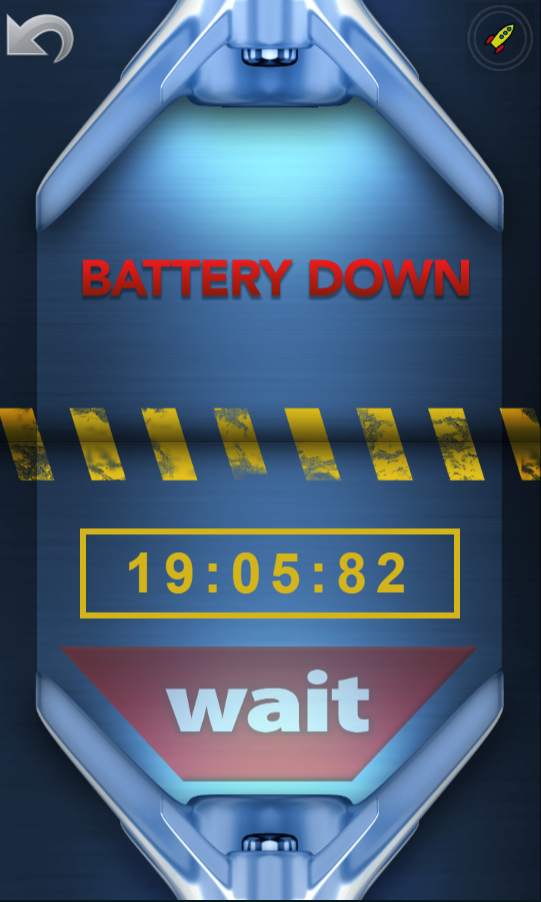The key to learning division facts is to learn them gradually. Students should work a few minutes at a time and then take a break. Rocket Math Online Game has students work for five minutes at a time (although the teacher can increase it to 10 or 15 minutes if the student wants it), and then the game pauses for a 20-minute break. Breaks will help keep students from becoming tired of the game and ensure they want to keep playing. Learning Math Facts is a marathon, not a sprint, so we want them to do Rocket Math once or twice a day for a few months. That’s how they will come to master the math facts.

Typically, students learn division in fourth or fifth grade, but they can learn it earlier if they understand the concept. Division isn’t harder than multiplication, but it will be if students have not mastered multiplication first. That’s why having students work through Set Z of multiplication before starting division is essential.

Here are the Learning Tracks offered by Rocket Math Online Game:

• The Basic Learning Tracks
• 3. Multiplication 1s through 9s (priority)
• 4. Division 1s through 9s (secondary priority)
• The Alternative Learning Tracks: learning multiplication and division facts in families
• 11. Fact Families (x,÷) to 20
• 12. Fact Families (x,÷) from 21
• Optional Learning Tracks
• 9. Multiplication 10s-11s-12s
• 10. Division 10s-11s-12s

When they have mastered multiplication and the 1s-9s of division, students can go on to Multiplication 10s-11s-12s and Division 10s-11s-12s.

As noted above, learning by fact families is an alternative route to learning basic multiplication and division facts.  The first Learning Track would be #11 Fact Families (x,÷) to 20 followed by #12 Fact Families (x,÷) from 21.

## Rocket Math Online Game – The Best Tool to Learn Math Facts

Mastering the basic facts in addition, subtraction, multiplication, and division will not only help students succeed in school, but are essential skills to have outside of the classroom. If you want your students to be successful at math and enjoy learning, memorizing these math facts is vital. With Rocket Math Online Game, your students will be engaged and excited to play. Students will be able to see their progress, celebrate their wins, and take pride in what they learn. It doesn’t take much to motivate your students, just a sincere recognition of their achievement. They will know when they have accomplished something, and if you recognize it as well, then they will feel proud of themselves.

There’s a free two-week trial of the Rocket Math Online Game so you can see for yourself how well it works and how students love it.

## The Ultimate Guide to Math Fact Fluency

Students counting on their fingers is a sure-tell sign that they didn’t acquire math fact fluency. It is sad to see students, ashamed of the only thing they know, counting on their fingers under their desks. Our elementary educational mission is failing students who haven’t developed math fact fluency, which  is the foundation to more advanced math skills.

Developing math fact fluency takes structure, organization, and work on the part of both teachers and students. In this article, I will share everything you need to know about developing math fact fluency.

## What is Math Fact Fluency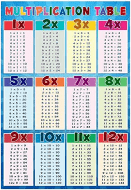Math facts are single-digit problems such as 7+9 or 6×8 or 14-5, and so on. A common name for all the multiplication math facts is the “multiplication table.” Math fact fluency is the ability to answer all math fact questions instantly from recall without having to think through the problem.

Students should be able to recall math facts instantly without having to count on their fingers or  hesitate to think about the answer. This may seem like a high bar, but our brains are great at recalling an unbelievable amount of information daily, and with practice, math facts can be recalled the same way.

## Three Reasons Why Math Fact Fluency is Important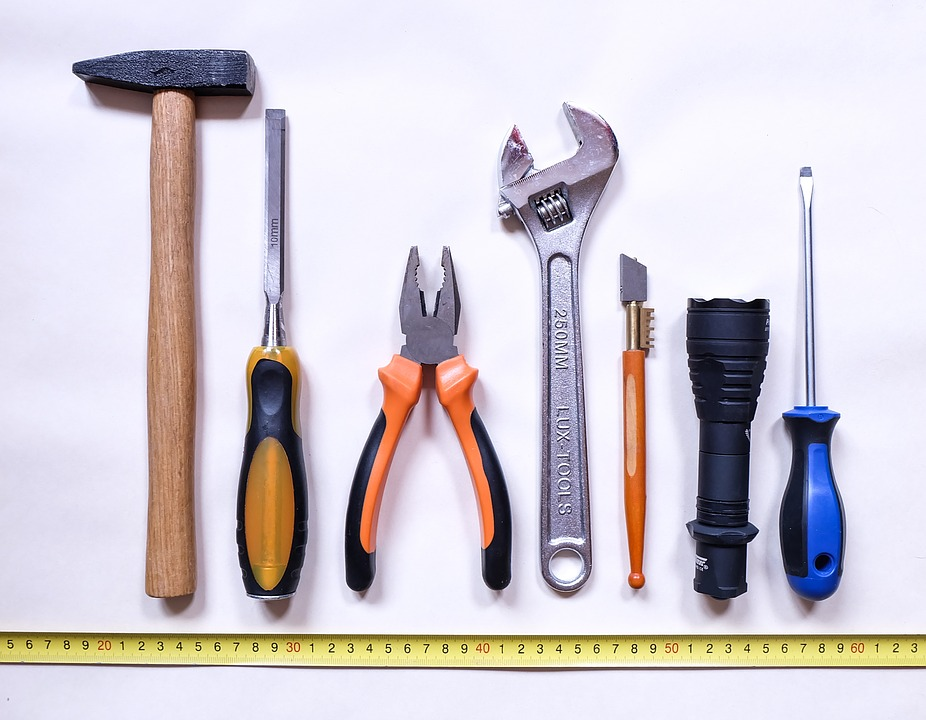Math fact fluency is critical because it is a “tool skill.” Meaning, it is a tool that is used in the process of doing other math problems. Developing this tool skill makes learning math easier as concepts get more complicated. This tool skill needs to be automatic in the student’s brain, in order to save precious short-term memory resources.

Math fact fluency can be compared to reading. Students must recognize words automatically to comprehend the author’s meaning. Otherwise, they will spend too much time decoding individual words.

When students are fluent in math facts, they are focused on the math process as a whole, rather than stopping to puzzle out the facts. This is important for three reasons:

### 1. Students with math fact fluency make fewer errors

Students who lack math fact fluency often make careless errors doing arithmetic computations . If they devote too much energy to deriving math facts, they lose sight of the problem at hand and make mistakes that would otherwise be obvious. Those who can effortlessly recall math facts can concentrate on what they are doing, and ultimately make fewer errors.

### 2. Math fact fluency makes learning math easier

When a new math procedure is introduced, students who have math fact fluency can easily follow the thread of instruction. Without this fluency, students fall behind instruction or demonstrations as they try working out math facts. This distraction takes away from a student absorbing all of the details necessary to successfully learn new math processes.

The first teacher to use Rocket Math to teach subtraction facts to her second graders realized the benefit first hand. She told me that with Rocket Math, she was able to teach regrouping in subtraction in just three days.

Her students mastered the math facts, and the outcome was extraordinary. The teacher shared that since these students had developed fluency in subtraction facts, they were able to learn other procedures easily.

### 3. Students who have developed math fact fluency enjoy math and always complete their work

Having to count on your fingers or look up facts on a timetable is slow and onerous. When students can’t work quickly, math problems become a dreaded drudgery. Students are motivated by mastering new skills, which will help them work faster and build confidence . Those who can quickly recall math facts will complete their work with ease , and enjoy the feeling of accomplishment.

## How to Build and Improve Math Fact FluencyBuilding and improving math fact fluency requires a systematic effort over the elementary years. It is a long climb to achieve mastery and there are no short-cuts.

Consistent daily practice throughout elementary school is important for retention. Slow and steady wins the race when building math fact fluency.

Math fact practice should be structured in such a way that students are learning a small number of facts at a time. These small groups of facts  should be practiced daily until students have reached mastery. As time goes on, more groups of math facts are introduced systematically in small amounts for students to master.

Learning 1s to 9s facts in the four basic operations will take elementary students months to master. Worksheets and game applications are two of the best ways to teach math fact fluency over time. Combining structured math fact learning, practice, and evaluation with fun math fact games helps students develop number sense and understand complex numerical relationships.

## Teaching Math Fact Fluency with Worksheets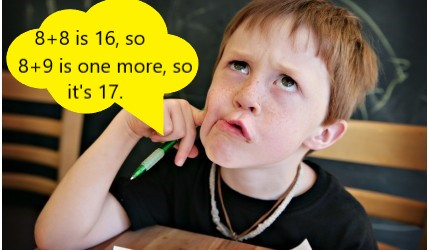Worksheets are popular tools that teachers reach for when teaching math facts, but sadly, they often fall short for the majority of students. A few select students will begin memorizing the facts on their own accord in order to make the worksheets easier, but most students will continue to slowly work out the facts either on their fingers or in their heads. These students may never develop a strong recall of the facts and become flustered when asked to answer problems on the spot.

Fortunately, there are specific worksheets that are effective in building math fact fluency. The key is having worksheets that are structured, systematic, and sequenced. Each worksheet should only have two to four facts to be learned.

By working on only two to four facts, these worksheets help teach memorization for a strong recall, rather than reinforcing working out problems slowly. Students  will then be able to remember these small groups of facts easier, and by the end of the worksheet will be writing answers from memory.

## Teaching Math Fact Fluency with The Rocket Math Worksheet Program

Rocket Math Worksheet Program improves upon this concept by using paired practice and saying facts aloud. Students partner up and practice quickly recalling facts together. One student asks the questions and watches for when their partner hesitates to answer. He or she then gives his or her partner more opportunities to practice the harder facts.

The students switch roles, and after both have answered questions, they then take a one minute test on the facts that they have learned so far. If students are answering as fast as their fingers will carry them, then they pass the level and move on to the next worksheet in the sequence.

Ten minutes of practice  every day gets the job done, especially when paired with using these facts in higher level math problems.

## Teaching Math Fact Fluency with Games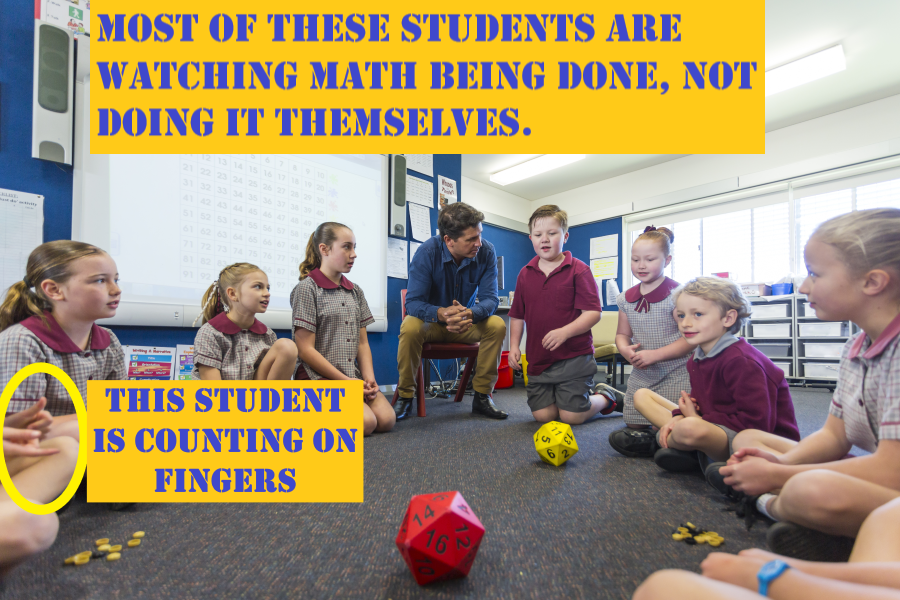In addition to worksheets, schools of education tell teachers to use games to “teach” math facts. Unfortunately, most games and fun activities do not actually help individual students learning math facts to the level of fluency. These games, such as bingo or dice, have several fallouts:

• Students spend most of their time waiting for their turn rather than practicing facts.
• They do not focus on teaching a small group of facts in a manner that helps students commit them to memory.
• The games do not adjust to an individual student’s level of fluency.
• Students can pace the game slowly enough to have time to figure out facts rather than requiring recall.
• It is difficult to keep every student engaged, as those who are behind are less likely to participate.

## Using the Rocket Math Online Game as an Effective Way to Teach Math Fact Fluency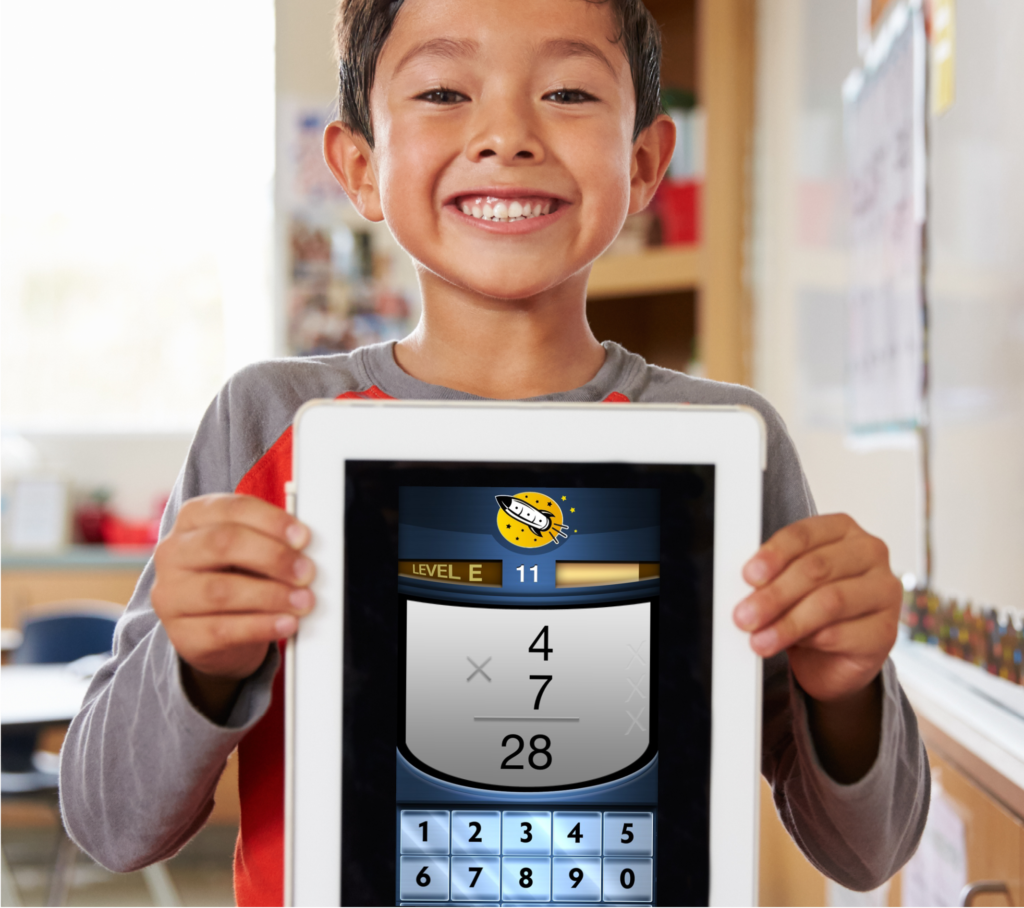There are games that are very effective at building math fact fluency. Games such as the Rocket Math Online Game have several important features that make a big difference.

1. Every student is engaged in answering math facts—not waiting for a turn.
2. Students learn only a few new facts at a time so that they can remember them.
3. The game provides lots of focused practice on each set of facts.
4. The game requires students to answer quickly, which guarantees the students recall the answer rather than “figuring it out” over and over.
5. The game gives an immediate correction and extra practice on any facts that students cannot answer quickly and correctly.
6. The game only introduces new facts once students demonstrate mastery of facts learned so far.
7. The game gives students explicit feedback so they have a sense of accomplishment as they work their way through an operation.

## Math Fact Fluency Benchmarks

The following benchmarks are reasonable expectations for a school that has an effective math fluency program in place. Of course, a student cannot write math facts any faster than they can normally write, so take that into account when looking at fluency benchmarks. Adjust the benchmarks for students who do not write quickly.

### Kindergarten Numeral Writing Fluency Benchmarks (digits)

Start of Year Mid-Year End of Year
20 digits per minute 40 digits per minute

### First Grade Numeral Writing Fluency Benchmarks (digits)

Start of Year Mid-Year End of Year
40 digits per minute 60 digits per minute 60 digits per minute

### First Grade Math Fact Fluency Benchmarks (problems per minute)

Start of Year Mid-Year End of Year
Addition: 12 per minute Addition: 25 per minute Addition: 25 per minute

### Second Grade Math Fact Fluency (problems per minute)

Start of Year Mid-Year End of Year
Addition: 25 per minute                      Addition: 30 per minute                      Addition: 30 per minute
Subtraction: 12 per minute Subtraction: 25 per minute

Third Grade Math Fact Fluency Benchmarks (problems per minute)
Start of Year Mid-Year End of Year
Addition: 30 per minute Addition: 30 per minute Addition: 30 per minute
Subtraction: 30 per minute Subtraction: 30 per minute Subtraction: 30 per minute
Multiplication: 30 per minute Multiplication: 30 per minute

Fourth Grade Math Fact Fluency Benchmarks (problems per minute)
Start of Year Mid-Year End of Year
Addition: 35 per minute Addition: 35 per minute Addition: 35 per minute
Subtraction: 35 per minute Subtraction: 35 per minute Subtraction: 35 per minute
Multiplication: 35 per minute Multiplication: 35 per minute Multiplication: 35 per minute
Division: 20 per minute Division: 35 per minute

Fifth Grade Math Fact Fluency Benchmarks (problems per minute)
Start of Year Mid-Year End of Year
Addition: 35 per minute Addition: 40 per minute Addition: 40 per minute
Subtraction: 35 per minute Subtraction: 40 per minute Subtraction: 40 per minute
Multiplication: 35 per minute Multiplication: 40 per minute Multiplication: 40 per minute
Division: 35 per minute Division: 40 per minute Division: 40 per minute

Math Fact Fluency Assessment

Use this printable packet of free math fact fluency assessments to test your students’ skill levels relative to  the above benchmarks. This will give you a clear idea of your students’ fluency and where there is room for opportunity.

Rocket Math’s assessment packet includes a writing speed test, which helps create realistic expectations for individual students. Using the goal sheet ensures you will evaluate the individual student math fact fluency in light of their writing speed.

Rate students as:

1. Weak, needs fact work
2. Good, but fact work could help
3. Strong, fact work not needed

Special triage priority: if you have fourth-grade students and above, start with multiplication facts. Multiplication facts are essential to future success in math above fourth grade. Even if fourth graders are counting on their fingers for addition and subtraction, teach multiplication mastery first. If fourth graders move to the next grade without strong multiplication fact fluency, they will have a hard time successfully progressing through math.

## The Best Tools for Developing Math Fact Fluency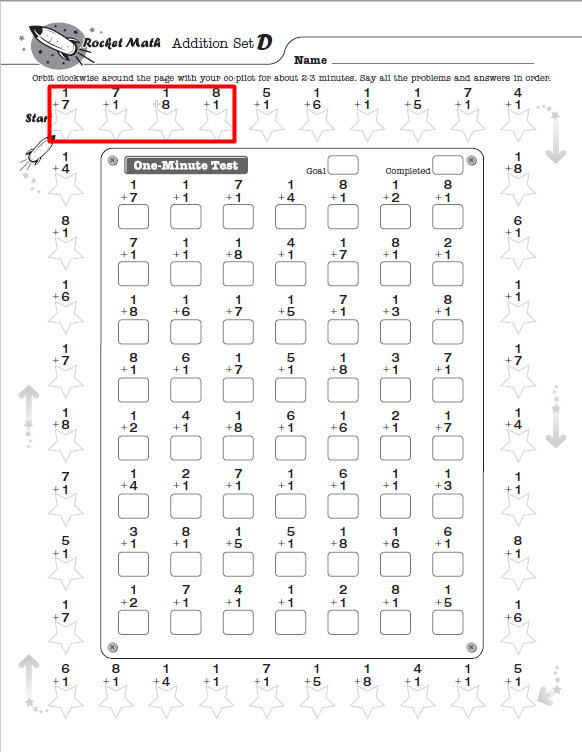With the right tools, any student can develop math fact fluency and have fun while doing it! Students use Rocket Math’s Subscription Worksheet Program to practice with partners, then take timed tests. Rocket Math also offers math facts practice online through the Rocket Math Online Game. Students can log in and play from any device, anywhere, any time of day! Start a free trial today.

Both the worksheet program and the online game help students master addition, subtraction, multiplication, and division math facts for a lifetime of success in math.

## Positive Praise: Building Effective Teaching Habits

Positive praise is one of the most effective ways to encourage wanted behaviors from students. Because building habits is not an easy task, here are a few things you can do to start easily incorporating positive praise in the classroom.

1. Be prepared with positive phrases
2. Develop the most effective wording
3. Start Small with two areas you would like to see improved behavior
4. Practice in the Classroom and watch the effect it has on your students
5. Grow and expand your positive phrases over time as you master the habit

## Be Prepared with Positive Praise Phrases

I distinctly remember trying to help pre-service teachers build the teaching habit of positive praise. I would make suggestions and then observe. Trying to implement my suggestions wasn’t as easy as you would imagine – these teachers would glance in my direction and start the sentence “I like the way you’re . . .” and then trail off without knowing what to say.

Teachers want to use positivity and affirmation with their students, however, in my experience, they don’t always have the appropriate words ready to praise good behavior. Building the teaching habit of positive praise starts with getting the right words ready.

Recently I was reminded of this key component of building the new habit of making more positive statements. I wanted to personally develop this positive statement habit, but for some reason was not making the progress I had hoped for.

I quickly realized that I was making the same mistake I had watched the pre-service teachers make. I was unable to make more positive statements because I did not have any in mind that were ready-to-use.

To build the habit of making more positive statements, I would have to start memorizing some key phrases to keep on standby, ready to use when I needed them.

## Positive Praise Example Phrases: How to Develop the Right Wording

The first step in positive praise is learning and developing the most effective wording. Using effective wording means you are getting through to your student, and clearly communicating that you appreciate the good behavior they are exhibiting.

Praise is most effective when it is prompt – when you deliver the praise in the moment. Can you picture a specific scenario in your classroom when many of the students are not doing as you asked, while a few students are dutifully following instructions?

This is the perfect scenario to use positive praise not only in rewarding students with good behavior but also encouraging other students to follow suit. Don’t be afraid to praise good behavior loud and proud for the rest of the classroom to hear!

Here are some examples of positive praise:

• Look at Alan so smart sitting in his seat and showing me he is ready to learn. Way to go, Alan.
• I see Beto is tracking with his finger while Claudio is saying the facts. That’s the way to help your partner!
• Julia, you are so sharp having your eyes on the teacher, so you can learn!  I am impressed.
• Stacy and Sophia know just what to do, they have their books open to page XX.  They are so on top of it!
• Fantastic, Justin! You put your pencil down and are waiting for directions.  I can tell you’re going to college.
• Stephanie is being such a great on-task student by working quietly and not talking.

## Start Small: Pick Two Key Behaviors You Would Like to See More Of

Start out by choosing wanted behaviors from the two most annoying or frustrating scenarios you face as a teacher.  Stating small will help you build a consistent habit of giving positive praise.

Take these two wanted behaviors and build two praise statements you can easily use in-the-moment. Make sure the statement names the behavior specifically. Always include the student’s name, and keep it simple and affirmative.

Now, take a note card or piece of paper and write down these two statements. Don’t wait! Write them down now and keep this note in front of you while you teach. It will serve as a reminder throughout your day to incorporate positive praise as much as possible.

Practice saying these phrases aloud until you have them memorized and can recall them without having to think about it. The most important step in building this habit? Actually practicing positive statements in the classroom.

With these key components and diligent practice in the classroom, you will quickly build the habit of positively praising your students.

## Positive Praise in The Classroom: Will it Make a Difference?

Fortunately, positive praise is free and can be implemented at any time throughout the school year. Start using positive praise now, and watch how your students respond.

Prepare yourself for giving positive praise when you are about to begin those frustrating scenarios. When the activity begins, look for opportunities to praise the behavior you are looking for when you notice students who are off-task.

You will see results when you use positive praise genuinely and with enthusiasm. You will know it is working if you watch for those distracted students taking notice of who is being praised. If you notice this happening, keep it up. The more praise you give for wanted behavior, the more that behavior will occur.

## Grow and Expand Your Positive Praise HabitNow that you know how to promote a specific behavior with positive praise, you can systematically develop statements for all your troublesome areas.  Every time students are not doing what you want, think of what you want them to do instead.  Behavior analysts call those replacement behaviors.

Positive praise can also be used creatively alongside other motivational tools in the classroom. When I began my teaching career I was in the habit of scolding behaviors I did not want. Early in my career, I learned the effectiveness of positive praise and began incorporating it into my daily routine.

When I saw the behavior I wanted I would give loud and proud praise for all to hear. I decided to couple this by adding marbles to a jar every time I gave praise, as an added motivational tool – so students could see how well they have been doing. It worked wonders on increasing wanted behavior.

Building new habits is never easy, but I can personally say that as a teacher, learning to incorporate positive praise into your teaching routine will not only help students learn, but it will save you a lot of frustration!

## Beginning Numerals and Counting

Dr. Don has created another math program and put it into the Universal level virtual filing cabinet at Rocket Math. This is a beginning program for kindergarten students and is to help them learn counting and numerals. That means they can’t learn on their own, the teacher must provide instruction. Teachers can use the counting objects kindergarten worksheets to effectively teach students to count objects aloud and then match the word with the numeral. You can see the top half of Worksheet A above.

If you’re already a Rocket Math Universal Level subscriber, you can find the worksheet in your virtual filing cabinet. Not a subscriber yet? Get the counting worksheets.

## I Do: Demonstration of Counting

Each worksheet begins with a demonstration of counting objects and circling the numeral that matches. On Worksheet A, there are only the numerals two and three to learn. The teacher demonstrates (best with a document camera so all students can see) how she counts the objects and then points out that the answer is circled. Suggested teaching language is something like this,

“I can do these. Watch me count the frogs. One, two, three.. There are three frogs in this box. So they circled the three. Everybody, touch here where the three is circled. Good.

How many frogs were in this box, everybody? Yes, three.

Now watch me do the next box.”

## We Do: Counting Together

In the “We Do” portion of the worksheet, the teacher counts the stars first as a demo and then with the students. Worksheet A you all just count three stars. Suggested teaching language is something like this:

“Our ‘We Do’ says to touch and count. Start at zero and count each star.

We are going to touch and count the stars. Put your counting finger on zero,

everybody. We are going to start at zero and count each star. Let’s count.

One, two, three. We counted three stars. That was great!

Let’s do it again! Fingers on zero, everybody. Let’s count. One…”

By Worksheet S the teacher and the students are counting 12 stars together.

The program has a page of teacher directions with suggested language for teaching the worksheets.

## You Do: Independent Counting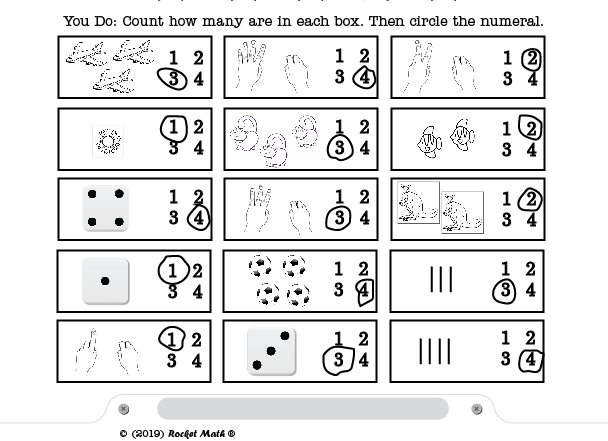In the “You do” portion of the worksheet (after learning the numerals with the teacher), the students are asked to count the items in each box and circle the correct number. They are not asked to form the numerals–that’s numeral writing skill. They just identify the numeral and circle it. Besides cute items, there are also dice to count, fingers to count, and hash marks to count–so students can learn multiple ways of keeping track of numbers.

Passing a level requires 100% accuracy. Students who make any errors should be worked with until they can complete the worksheet independently and get all the items correct.

## Rocket Math’s Counting objects worksheets for Kindergarten

This Beginning numerals program will build strong beginning math skills for kindergarten students learning the meaning of numerals. Combined with Rocket Writing for Numerals it will set students up for success in elementary math.

If you’re already a Rocket Math Universal Level subscriber, you can find the worksheet in your virtual filing cabinet [use your link]. Not a subscriber yet? Get the counting worksheets.

## Math Worksheets for Kindergarten, 1st, 2nd, 3rd, 4th grade+

Rocket Math worksheets are a great way to teach math facts to children of all ages – starting as early as Kindergarten when students begin learning how to read and write numbers. The Rocket Math Universal Worksheet Program is designed for daily practice in order to build a solid foundation of basic math skills.

Our Universal Worksheet Program follows a simple structure and routine to help students progress at an appropriate rate throughout their different grade levels. Throughout the sequence, students learn all of the building blocks necessary to succeed throughout elementary and middle school.

If you have students that are behind for their grade level, our worksheet program makes it easy for you to revisit previous lessons that will reinforce the concepts that are necessary to move forward. Likewise, there are plenty of supplemental worksheets within the program to keep advanced students engaged.

## Kindergarten Math Worksheets

The first math-related goal for Kindergarteners should be to learn how to write numerals. It is important for children to learn the most efficient way to write numerals. Think about it – how do you write the number eight? Where does your pen begin on the page?

Believe it or not, this is something that is learned and becomes second nature.  This skill is important to develop early on as the first building block to learning math.

Rocket Writing for Numerals is a 72-page program for students to learn how to write the numerals efficiently.  It proceeds from how to write numerals and goes until they can write 40 digits in a minute. It is part of the Rocket Math Universal Worksheet Program and is designed to be practiced on a daily basis.

## 1st Grade Math Worksheets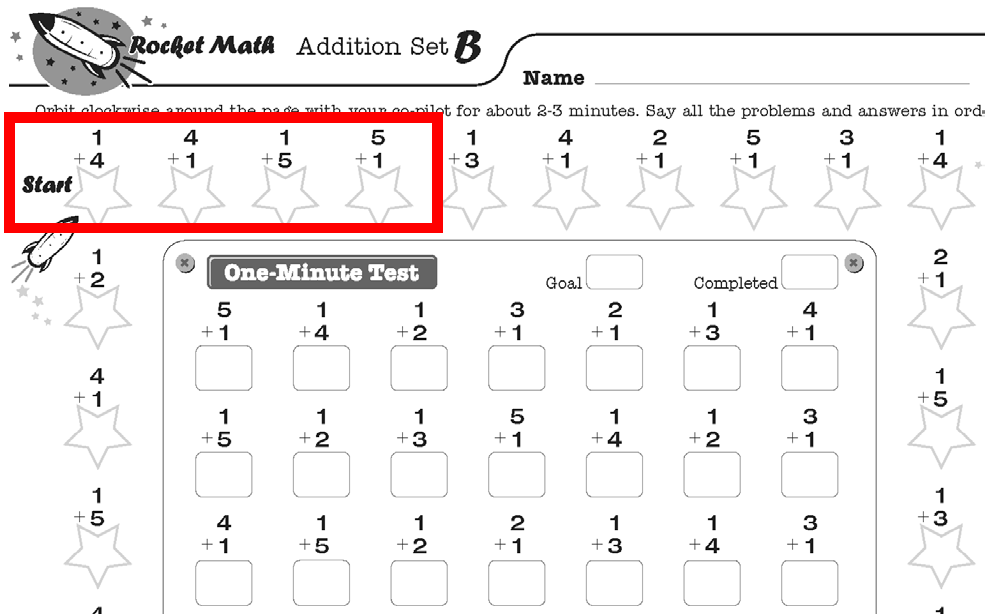If children in first grade cannot write numerals legibly and efficiently, they should begin the year with Rocket Writing for Numerals. Once they understand the concept of addition, first graders are ready to begin memorizing addition facts.

The Rocket Math Worksheet Program includes Addition 1s through 9s.  Students work through 26 levels (A to Z) learning two facts and their reverses on each level.  They practice orally for 2 minutes with a partner who corrects any hesitations or errors.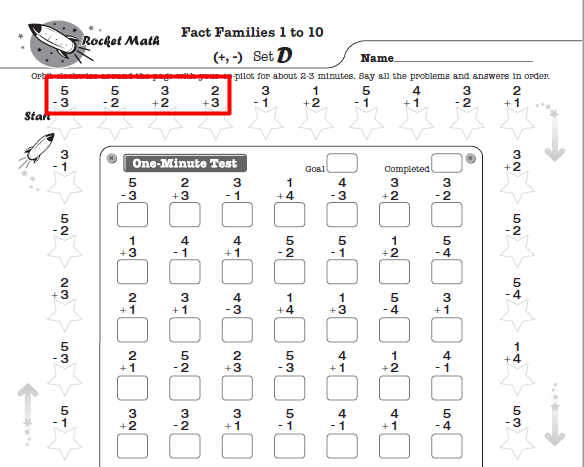Alternatively, once students have learned the concepts for both addition and subtraction, they can begin to learn Fact Families. Our Fact Families 1 to 10 Add and Subtract worksheet program begins teaching fact families. Set D of this worksheet to the right is an example, teaching four related math facts such as 3+2, 2+3, 5-2, and 5-3.

Common Core suggests that students be fluent with addition facts up to 20, such as 13+6=19. Personally, I think if a student knows 3+6=9, they don’t need to practice 13+6.  However, Rocket Math has made available a program for these facts, Add to 20 in the Universal subscription.

Rocket Math makes it easy for teachers, as the consistent structure provides an easy daily routine for students in all programs and levels. Because the Rocket Math Worksheet program follows a sequence and routine, it is easy for students to continue working together even when studying different programs or levels.

## 2nd Grade Math Worksheets

Upon starting second grade, students should have mastered all of their addition facts, or fact families 1 to 10. If they have not mastered those facts, you should begin with the first-grade worksheets (Addition 1s through 9s/Fact Families 1 to 10 Add and Subtract) until they are ready to move forward.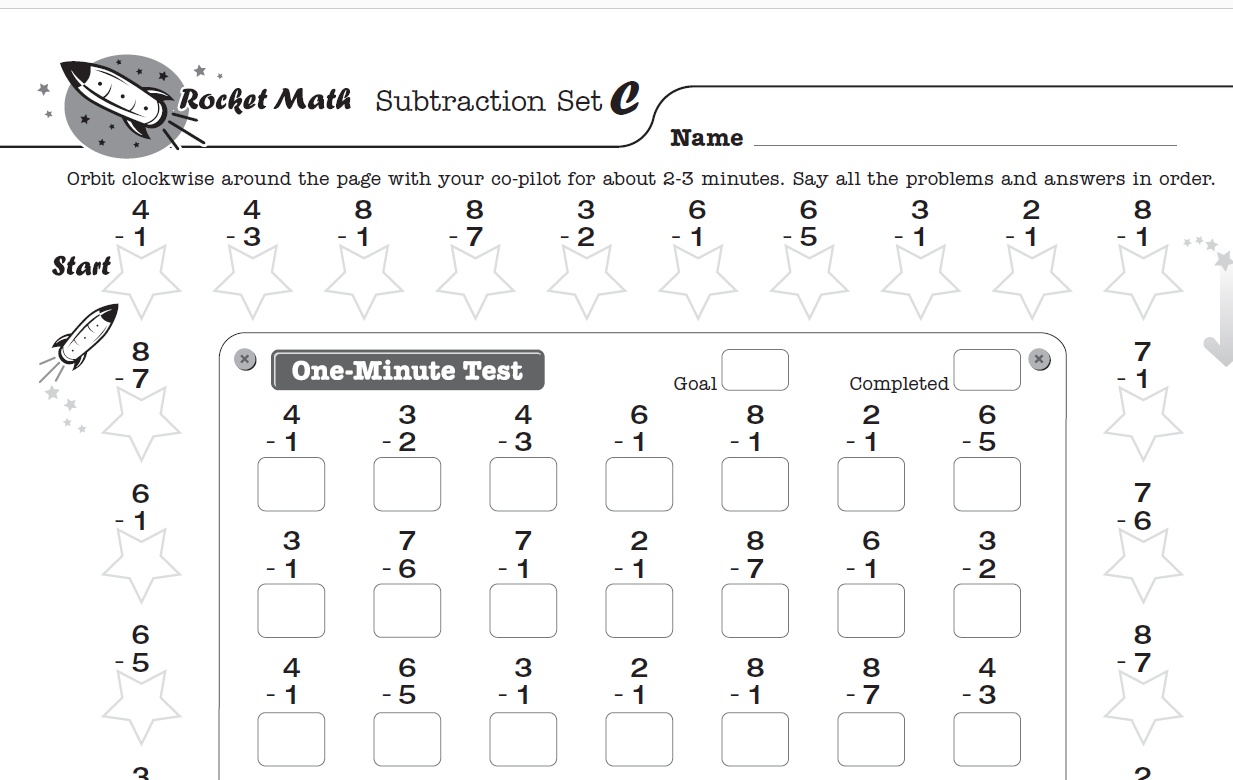The goal for second graders is to learn subtraction (and addition facts) by the end of the year.  Rocket Math has a Worksheet Program for Subtraction 1s through 9s that is perfect for second grade.  If students master the subtraction facts through practicing with Rocket Math they will be able to learn the lessons of re-grouping, a.k.a “borrowing”, much more easily.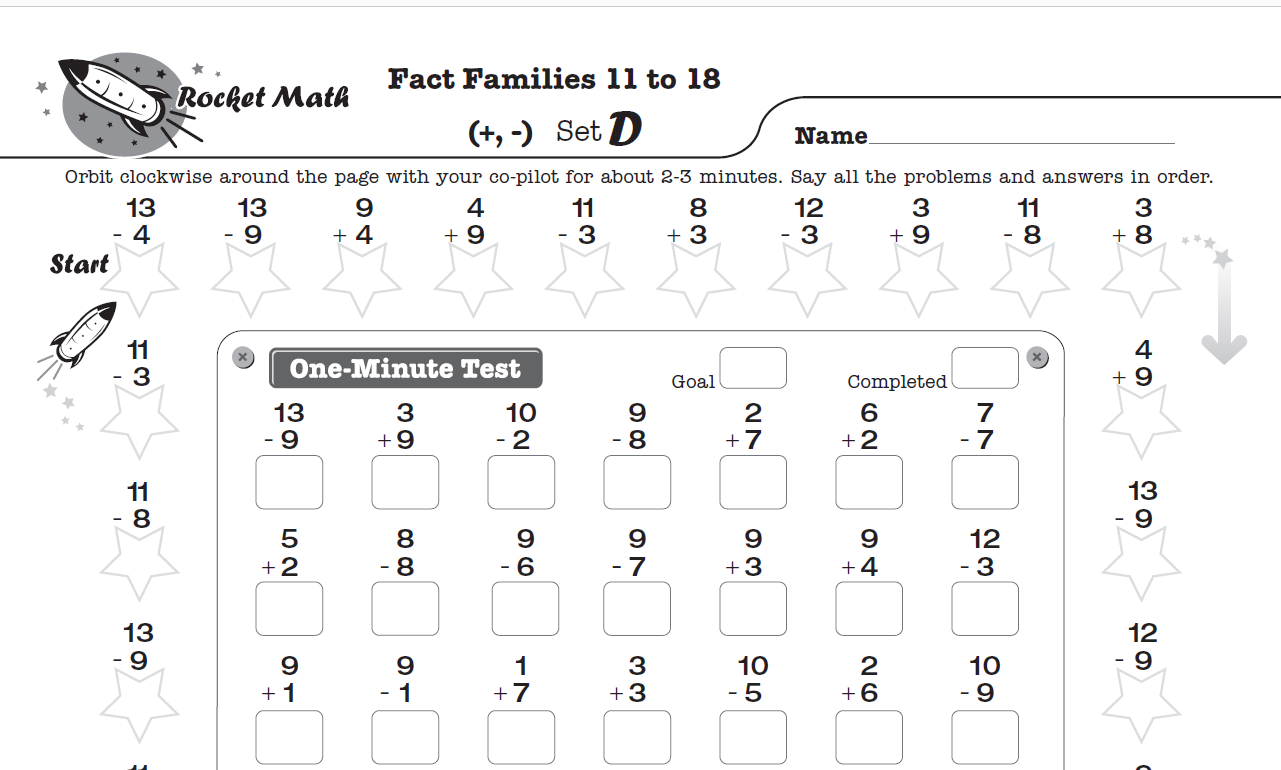If students have been using the Fact Families Program, it is best to continue using that sequence. Second graders should be ready for Fact Families 11 to 18 Add & Subtract. The last ten levels of this program review all the fact families so that students will be quite solid in their mastery by the time they reach Level Z.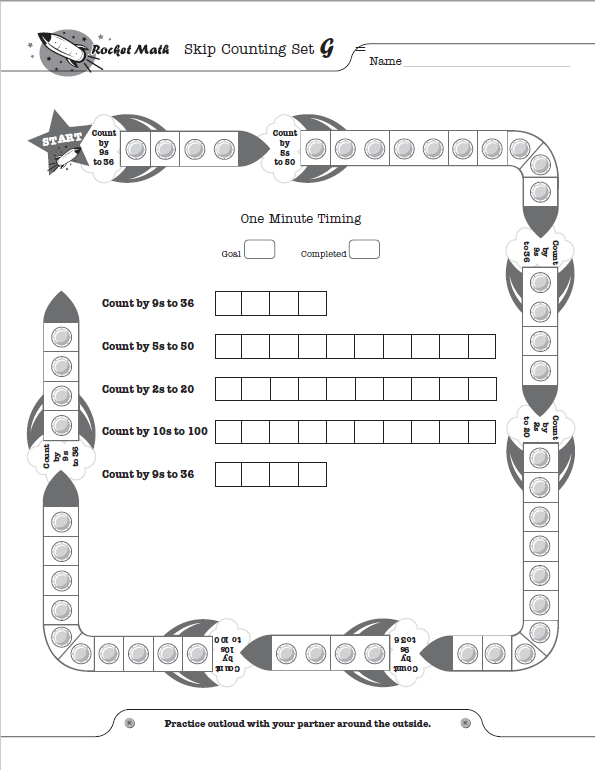For second-grade students who have mastered addition and subtraction facts, skip counting is a great next step. Skip counting is easy and fun for students while preparing them for multiplication in third grade.

Rocket Math’s Skip Counting program is a uniquely designed worksheet. When students are practicing together, the checker has to rotate the paper to keep up as the other is quickly skip counting.

Because of that, students especially enjoy this worksheet. Not to mention, the design incorporated playful Rocket graphics, which causes it to resemble a game rather than a math worksheet!

Common Core suggests that students be fluent with subtraction facts up to 20, such as 19-6=13. Personally, I think if a student knows 9-6=3, they don’t need to practice 19-6.  However, Rocket Math has made available a program, Subtract from 20, so students can practice these facts.

## 3rd Grade Math Worksheets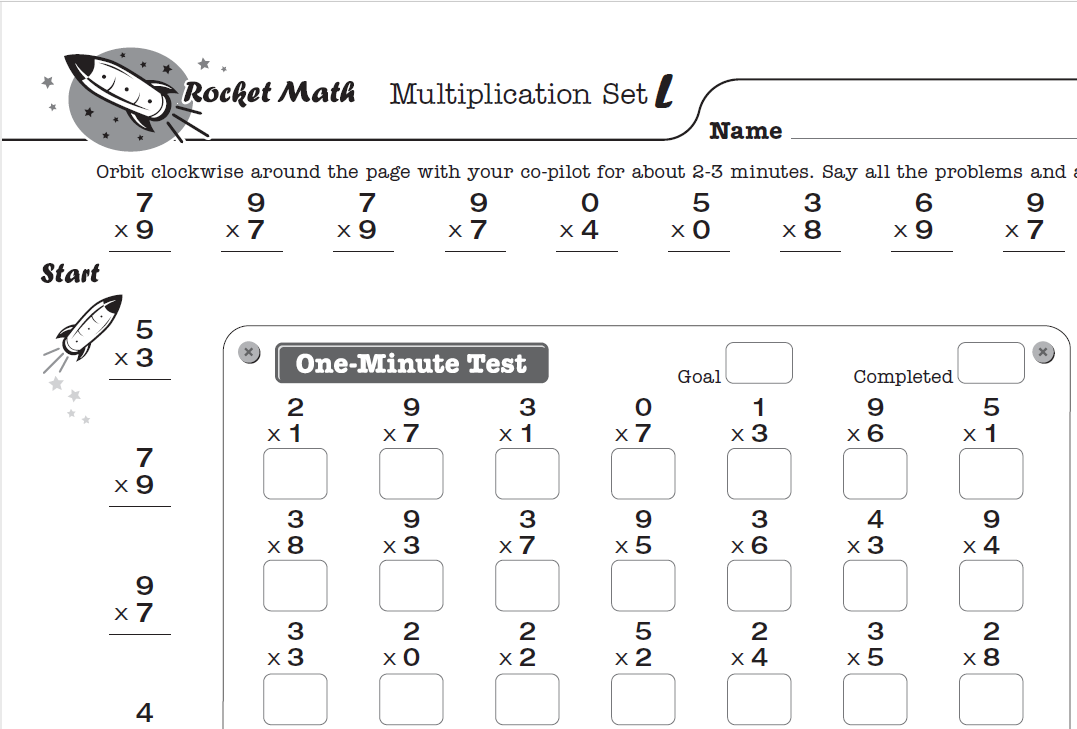The priority for third grade is to learn multiplication. Textbooks begin teaching the concept of multiplication from very early in 3rd grade.  Your goal should be to introduce multiplication facts by the time the textbook is giving students multiplication problems to solve.

Using the Rocket Math Skip Counting worksheet can help ease students into learning multiplication facts. If possible the Skip Counting worksheet should be used before students are asked to start performing a lot of multiplication.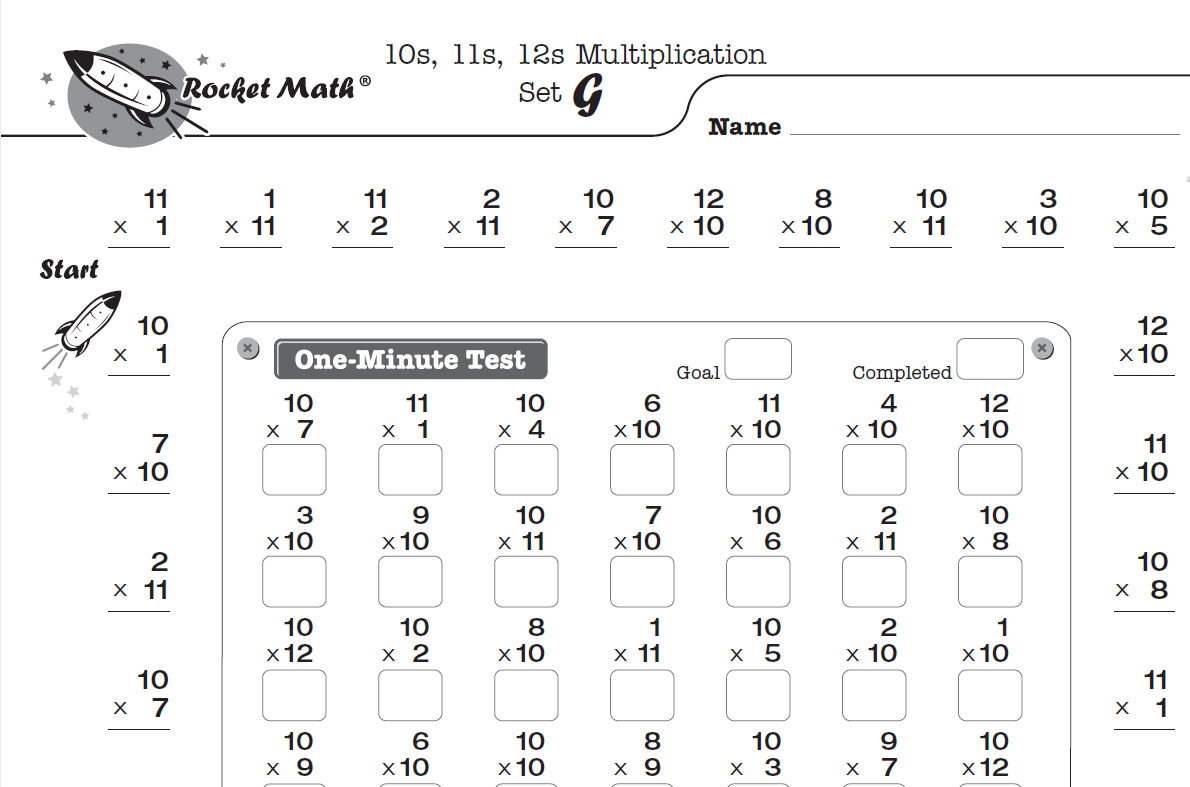The first step to successfully teaching multiplication facts is teaching memorization. The Rocket Math Multiplication 1s to 9s is designed to build strong multiplication fact fluency and recall. This technique avoids the problem of students having to look up facts in times tables, over and over again.

Achieving mastery in multiplication facts is the only way for students to keep up in math throughout elementary and middle school. Even if students come to you without having a solid foundation of addition and subtraction, it is crucial that you teach mastery in multiplication.

For quick-learning students, who get through Multiplication 1s through 9s before the end of the year, a good supplemental Worksheet Program is Multiplication 10s, 11s, 12s.  Building upon the facts from Multiplication 1s to 9s, students progress easily through this next set of facts.  And of course, students consider it a badge of honor to be members of the “tens, elevens, and twelves” club!

## 4th Grade Math Worksheets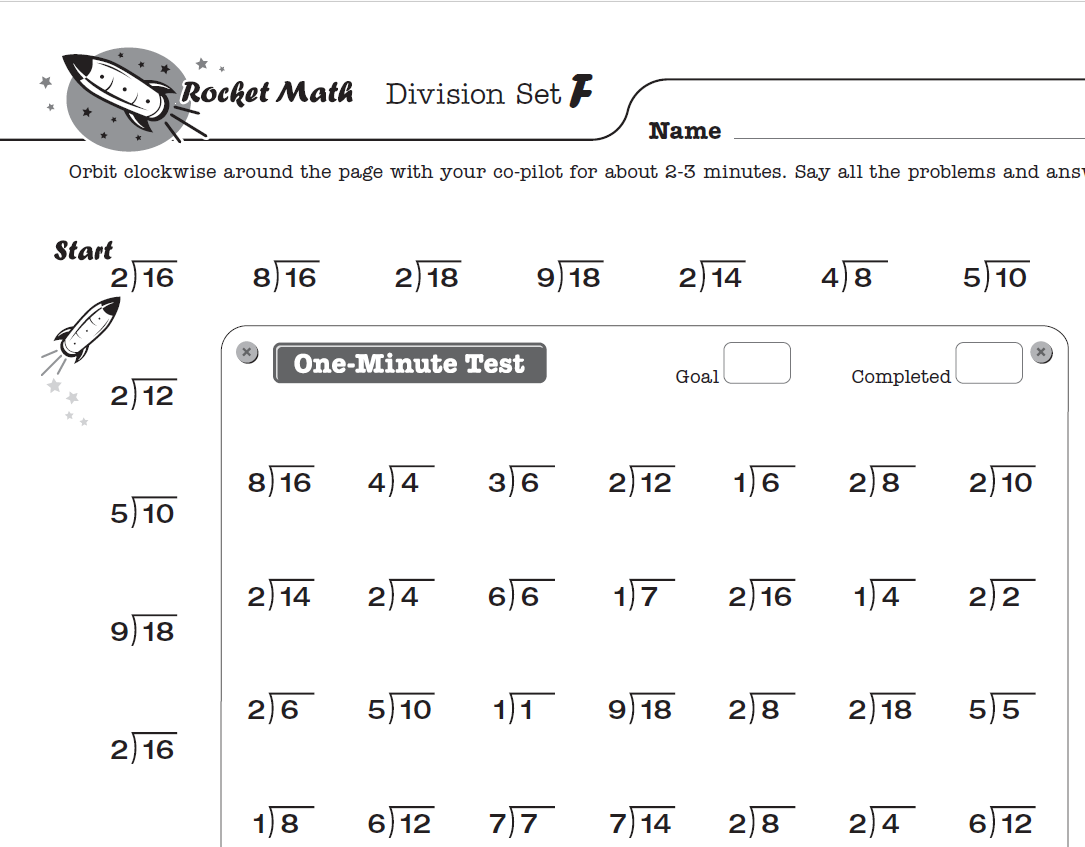By the end of fourth grade, students should have learned all four basic operations of math facts. Fourth graders should be using then Division 1s through 9s worksheet to learn division facts.

While division facts are derived from multiplication, it is very valuable for students to explicitly learn the division facts. Long division comes much easier for students who have learned division facts.

For students who get all the way through Level Z in basic division, there is a supplemental program: Division 10s, 11s, 12s.  It builds upon and reviews facts from Division 1s to 9s while keeping learning fun for students who work faster.

## Math Worksheets for 5th grade and up

Students who have been learning math facts since kindergarten have built a great foundation by the time they reach fifth grade.  Unfortunately, this is not the case for many students, which makes it difficult for teachers to create lessons suitable for everyone’s level.

Rocket Math provides an easy solution for these teachers. Simply take ten minutes a day utilizing the worksheet program to teach the four basic operations. While these students are catching up, the remaining students can do Rocket Math as well!

We offer five programs that teach more advanced math skills that follow the same structure and routine. Each one of these five different programs includes video lessons that teach students both how to use the program and how to do the skill that is being introduced.

• Teaches 100 fractions and what they are equivalent to.

2) Factors

• Shows how to find all the factors of a number, quickly and reliably.

3) Learning to Add Integers (positive and negative numbers)

• Uses a vertical number line to teach these problems.

4) Learning to Subtract Integers (positive and negative numbers)

• Uses the vertical number line to show how these work.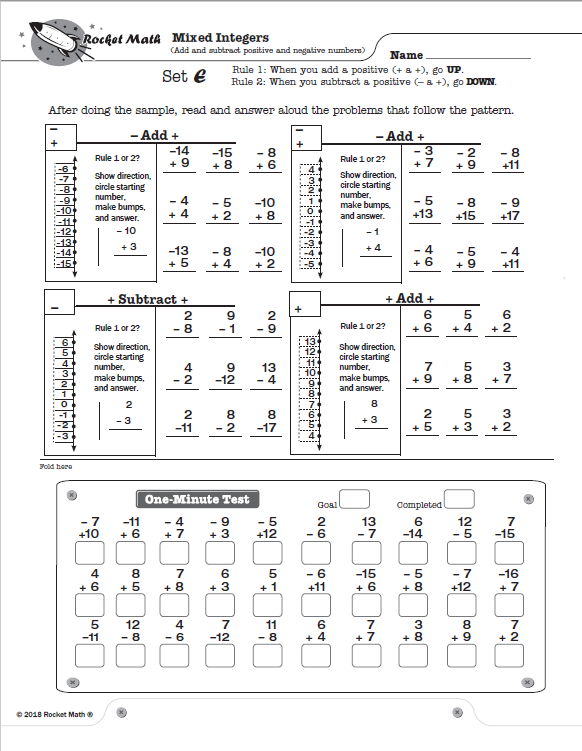5) Mixed Integers (adding and subtracting positive and negative numbers)

• Uses the same vertical number lines but now mixes the 8 types of problems together.

Rocket Math’s goal is to make learning fun for everyone – students and teachers. By following the worksheet program, even students who have struggled in the past can begin gaining confidence in their math skills.

With the right tools, students can enjoy learning math and teachers can relax knowing that a strong foundation is being built. Our FAQs page for Teacher Instructions and How to Implement the Worksheet program can help answer any questions you might have.

## The Ultimate Math Facts Test Online for Kids

You’re probably looking for a math facts test online to evaluate how well your students are learning their math facts. But did you know, it’s more effective to teach and test at the same time? Rocket Math’s online game does exactly that, making it the ultimate math fact online testing app.

## Testing without teaching doesn’t work

Too many math facts tests online spend a lot of time asking students facts they don’t know. As a result, students learn inefficient ways to solve math fact problems, like counting on their fingers.  Even puzzling out answers from knowing doubles is tedious. Students will find memorization of math facts difficult if they constantly have to “figure out” math facts. Plus these two methods are slow, setting students up to fail timed math fact tests in class.

So what is the best way to test whether a student can recall math facts?

## Teach and test a small number of math facts in tandem

An effective math facts test online will start teaching as soon as the test finds the first math fact that the student can’t answer quickly.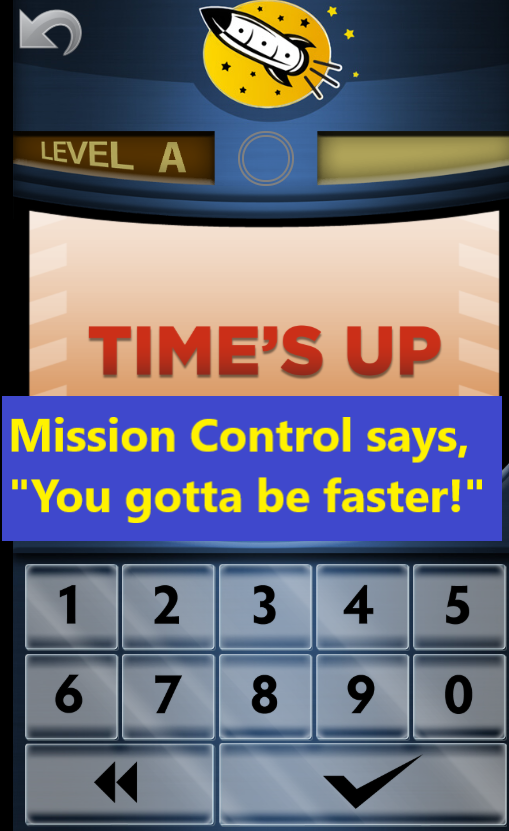It is important to begin teaching immediately for several reasons.

(1) Students think that they will always have to compute math facts on their fingers or with a number line.  They don’t know that they can and should get to a point where they instantly recall the answers to facts.  Helping students memorize a previously unlearned math fact immediately after they miss the problem shows them that they can successfully master any fact.

(2) Students need to know that we do NOT want them endlessly figuring out math facts.  By responding immediately with teaching, we send the message, “Hey, you didn’t know this fact instantly.  That’s not what we want.  Let’s practice this one, right now, so you can learn it.”  Requiring the student to answer the fact again faster reinforces the message.

(3) Teaching a fact works best when the fact is surrounded by facts the student already knows. Therefore, the best time to teach is when a student meets the first math fact they can’t instantly recall. An effective test online will mix the unrecallable math fact with a sea of already mastered material, teach the math fact, and then test it again.

(4) The student learns the difference between memorized and unmemorized facts. This helps the student understand that the goal is to instantly recall that fact.

(5) With a combined teaching and testing approach, a student’s success rate will be high since the student primarily answers facts they already know. As a result, students are motivated to learn more math facts.

## Why testing and teaching is the perfect learning paradigm

If your teaching program works carefully in sequence, students will encounter opportunities to practice facts they know (a good thing!).  Gradually, new facts will be introduced, practiced and tested. That is the perfect recipe for successful learning.  Over time, students will be able to master all the facts as the program works through its sequence.  Now you have accomplished the end goal: for students to learn the facts they didn’t know.  By testing and teaching as you go, students remain encouraged, learn the difference between problem-solving and memorization, and reach mastery.

## Rocket Math game: the ultimate math fact test onlineThe picture to the right shows me watching the very first kid try out the Rocket Math online game.  As soon as he saw math problems, he said, “I don’t really like math that much.”  But he saw the problem on the screen was 3 + 1 =, so he just typed in 4 because he knew the answer.  Then he saw 2 + 1 = and he says, “Well I know that,” and he typed in that answer.  The reverses (1 + 3 and 1 + 2) came up and he could answer those.  The online math app only introduced those two math facts and their reverses, but he had to answer twelve in a row to pass the test.

Over time, he became faster.  He answered all twelve math facts and received an on-screen congratulations. Mission Control (a fictional character in the math app) told him that he had “taken off with Set A” and could, “try for orbit” if he dared! After finishing the round, he brought the tablet over to me and said he liked it and wanted to play it again as soon as the mandatory 30-minute break was over.

Not only did the Rocket Math app teach and test four math facts in 5 minutes, but the student wanted to continue practicing math facts. That’s the beauty of a combined teaching and testing approach. Kind of a big deal, eh?

## Math Fluency Programs should be part of on-going elementary school routines

Most elementary teachers do some activities to promote math fluency.  Yet many elementary children are not fluent with math facts by the time they hit upper elementary or middle school.  A hit-or-miss approach allows too many students, especially the most vulnerable, to slip through the cracks.   Math fluency programs, like Rocket Math’s Worksheet Program, need to be part of your elementary school’s routine.  Effective math fluency programs should be properly structured and every math teacher should be on board, every year.

## Math fact fluency enables students to develop number sense

Many teachers learn in their training programs about the importance of “number sense.”  Students who have “number sense” can easily and flexibly understand relations between numbers.  They can recombine numbers in various ways and see the components of numbers.  Students with number sense can intuit the fact that addition and subtraction are different ways of looking at the same relations.

What is not taught in most schools of education is that developing fluency with the basic math facts ENABLES the development of number sense much better than anything else.  Once students memorize facts, they are available for students to call upon to understand alternate configurations of numbers. Students find it much easier to see the various combinations when they when they can easily recall math facts.  Once students master the basic facts, math games that give flexibility to thinking about numbers become much easier.

It may be hard for new teachers, straight from indoctrination in the schools of education, to imagine this is true.  However, if they land in an elementary school with a strong math fact fluency program they will see the beneficial effect of memorization.

##Why is math fact fluency important

In the primary grades, students who have not developed fluency in math facts will have a harder time learning basic computation.

Students who are not fluent with math facts find the worksheets in the primary grades to be laborious work.  They finish fewer of them and may begin to dislike math for this reason.

By the time students reach upper elementary, if they have not memorized the math facts, they find it very difficult to complete math assignments at their grade level.  They find themselves unable to estimate or do mental math for problem-solving.  The need to figure out math facts will continue to distract non-fluent students while they are learning new math procedures like algorithms.

In the upper grades, their inability to figure out multiplication facts becomes a huge stumbling block.  Manipulations of fractions, decimals, and percentages will not make intuitive sense to students because they haven’t memorized those facts.  Without math fact fluency, students rarely succeed in pre-algebra and may be prevented from learning algebra and college-level math entirely.

## Math fact fluency must be assured through regular monitoring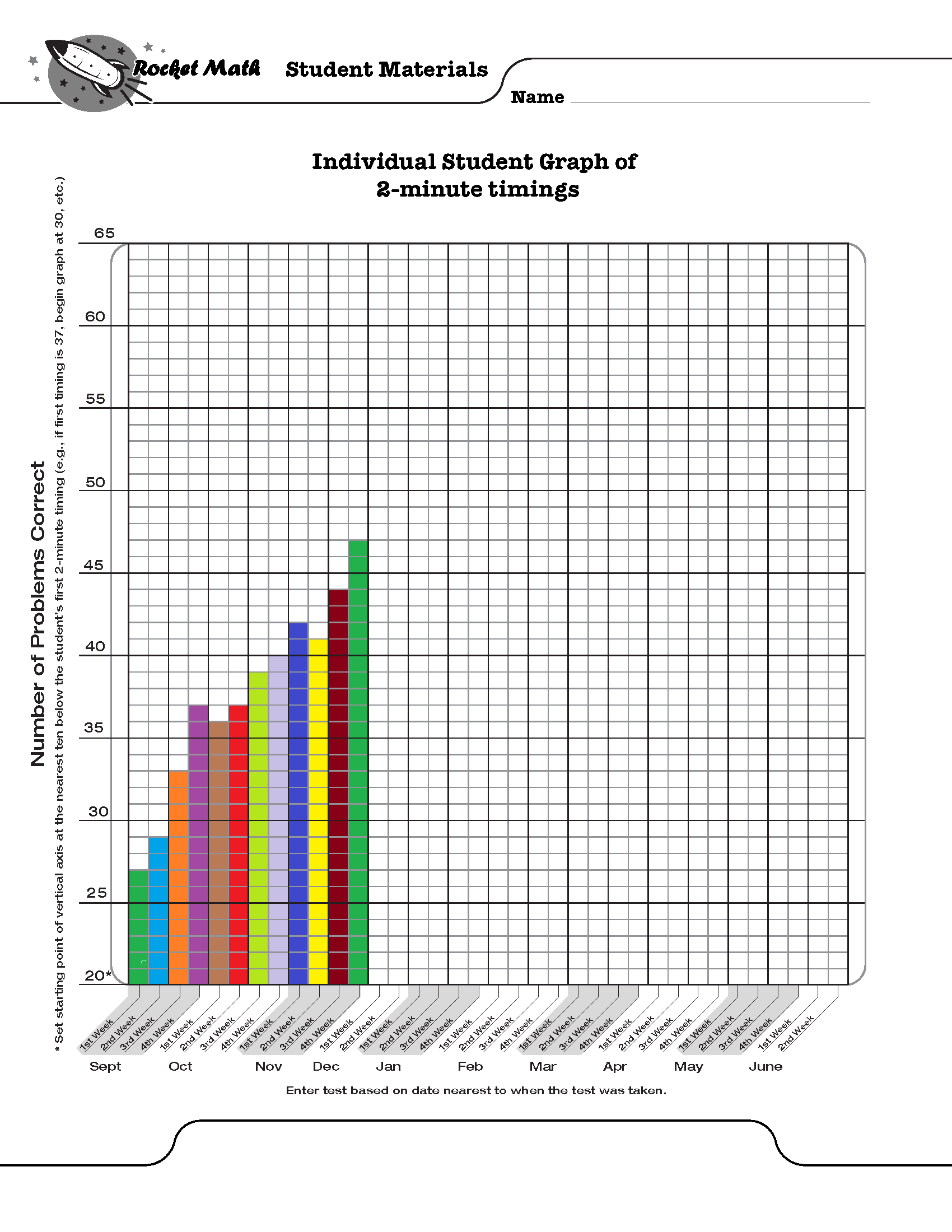Some students will need up to ten times more practice to develop math fluency than other students.  Therefore, monitoring student success in memorizing the facts is critical. Teachers can assume that what is “enough practice” for some students is NOT going to be enough practice for all students.  Effective math fluency programs must have a progress monitoring component built in.  Progress monitoring gives comparable timed tests of all the facts at intervals during the year.  Teachers look at the results of these timed tests to check on two things:

### 1. Are students gradually improving their fluency with all the facts gradually over the year?

In other words, are students able to answer more facts in the same amount of time?  If they aren’t improving, then the instructional procedures aren’t working and need to be modified or replaced.  Math fluency programs like Rocket Math’s Worksheet Program have two minute timings of all the facts in each operation that can be given and the results graphed to see if there is steady improvement.

### 2. Are all students reaching expected levels of performance at each grade level each year?

Proper math fluency programs identify students who are not meeting expectations and give them more intensive interventions.  Ultimately, by the end of fourth grade all students should be able to fluently answer basic 1s – 9s fact problems from memory in the four operations of add, subtract, multiply and divide. Fluent performance is generally assumed to be 40 problems per minute, unless students cannot write that quickly.

Expectations vary by grade level and the sequence with which schools teach facts can vary.  While it is great to achieve all that the Common Core suggests, it is critical only to assure that students master and gain fluency in 1s through 9s facts.  Some schools in some neighborhoods may find that waiting until second grade to begin math facts may not provide enough time for all students to achieve fluency.  When to begin fact fluency and how much to expect each year should be based on experience rather than some outside dictates.

## Successful math fluency programs must have these 3 features

1. ### Sequences of small sets

No one can memorize ten similar things, like the 2s facts, all at once. Students easily master math facts when they can learn and memorize small amounts of facts at one time. Effective math fluency programs define math fact sequences, which students memorize at their pace before moving onto new math facts. Rocket Math’s fluency program uses only two facts and their reverses in each set from A through Z.

2. ### Self-paced progress

Even if you only introduce small sets of math facts, some students need more time to memorize than others.  If you introduce the facts too fast, students will begin to jumble them together and progress will be lost. The pace of introducing facts must be based on mastery—not some pre-defined pace.  This is why doing all the multiplication facts as a class in the first six weeks of third grade does not work.  It is just too fast for some students.  Once they fall behind it all becomes a blur.

3. ### Effective practice and corrections

When students are practicing facts, they will come to ones they have forgotten or can’t recall immediately.  Those are the facts on which they need more practice.  Allowing students to stop and figure out the facts they don’t know while practicing, does not help the student commit them to memory.  Instead, students need to IMMEDIATELY receive the fact and the answer, repeat it and try to remember it.  Then they need to attempt that fact again in a few seconds, after doing another couple of problems.  If they have remembered the fact and can recall it, then they are on their way to fluency.  But students must practice the next day to cement in that learning.

Math fluency programs like Rocket Math’s Worksheet Program teach students math facts in small sets, allow students to progress at their own pace, and support effective practice and error correction. Each Rocket Math Worksheet program has 26 (A to Z) worksheets specially designed to help kids gradually (and successfully) master math skills. Gain access to all of them with a Universal Subscription or just the four basics (add, subtract, multiply, divide–1s to 9s) with a Basic Subscription.

## Just playing a math facts game won’t build math fact fluency

There are a lot of apps out there that look like they would help your child learn math fact fluency.  If they have to answer math facts, won’t that work?  Not really.  Just playing a game that asks you to answer facts won’t help you learn new facts.  In fact, most apps for practicing facts are discouraging to students who don’t know their facts well.  Why?  Because most of the people designing the app don’t have any experience teaching.  A teacher, like the creator of the Rocket Math App, is trained to effectively teach new math facts (or any facts) to a student and knows an effective math app from an ineffective one.

## 3 essential features of an effective math fact app

There are plenty of ineffective math apps.  Some apps don’t give the answers when a student doesn’t know them.  Some apps just fill in the answer for the student and then move on.  When the student doesn’t know the answer, the app has to teach it.  To teach math fact fluency, the app has to do these three things:

3. ### It has to ask the problem again after a short delay to see if the student can remember the answer.

Without doing these three things there’s no way the app is going to be able to teach a new fact to the student.

## An effective math app will only teach a few math facts at a time

Nobody can learn a bunch of new and similar things all at the same time.  A person can only learn two, three, or four facts at a time. You cannot expect to learn more.*  That’s enough for one session.  The student has to practice those facts a lot of times to commit them to memory.  Once or twice is not enough. It also won’t help to practice the same fact over and over.  Proper math fact fluency practice intermingles new math facts along with facts the learner has already memorized.  However, no more than two to four facts should be introduced at a time.  If a student has to answer a lot of random untaught math facts, you will have a very frustrated learner.

## Practice must focus on building math fact fluency

Some students learn to solve addition problems by counting on their fingers.  That’s a good beginner strategy, but students need to get past that stage. They need to be able to simply and quickly recall the answers to math facts. An app is good for developing recall.  But the app has to ask students to answer the facts quickly, faster than they can count on their fingers.  The app has to distinguish when a student is recalling the fact (which is quick) from figuring out the fact (which is slow).  Second, the app must repeatedly ask the learned facts in a random order, so students are recalling.  But the app should not throw in new facts until all the facts are mastered and can be answered quickly.

## Introduce new facts only when old facts are mastered

The trick to effectively teaching math facts is to introduce new math facts at an appropriate pace.  If you wait too long to introduce math facts, it gets boring and wastes time.  If you go too fast, students become confused.  Before introducing new facts, students need to master everything you’ve given them.  An effective app will test whether students have mastered the current batch of math facts before introducing more facts.  And it will also introduce math facts at a pace based on student mastery.  That’s the final piece of the puzzle to ensure students learn math facts from an app.

*Rocket Math App focuses on two facts and their reverses at a time, such as 3+4=7, 4+3=7, 3+5=8 and 5+3=8.

## Why Multiplication Games Are Awful & What to Do About It

As a university supervisor of pre-service teachers, I’ve seen my share of bad lessons.  Among the most painful were when student teachers would try to liven up their lessons to impress me by having the students do a math game.  My student teachers wanted their students to learn math facts and to do so in a fun way.  The picture above is typical of what I would see.  Here are the reasons that most multiplication games that the student teachers implemented were awful.

(For multiplication games that work in and out of the classroom, check out Rocket Math’s Worksheet Program and Online Game.)

## Waiting for your turn at a multiplication game is not learning!

As you can see in the picture above, all but one of the students are just waiting for their turn.  They aren’t doing math.  The students are just watching the student who is playing.  No one likes waiting, and your students are no exception.  Any game that has turn-taking among more than two students wastes time.

Make sure your multiplication games are structured so all or most students are engaged and playing all the time.  You want students to have as much engaging practice as possible while practicing math facts at speed.  If everyone can be doing that at the same time, that’s optimal.  No more than two students should be taking turns at a time.

## A multiplication game that allows using a known strategy to figure out facts (like finger counting) is not learning!

Learning math facts involves memorizing these facts so that students know them by memory, by recall.  Committing facts to memory is why there is a need for lots of practice.  If the game allows time for students to count on their fingers or use some other strategy for figuring out the answer to facts, then there is no incentive for students to get better.

In the lower left corner of the picture you can see one student counting on their fingers—which is better than just watching—but is not learning the facts, it is just figuring them out.  The most able students in an elementary school are able to memorize facts on their own when they tire of figuring them out day in and day out.  But the rest of the students will just do their work patiently year after year without memorizing if you don’t create the conditions for them to memorize facts.

Make sure that your multiplication games reward remembering facts quickly rather than just figuring them out.  Speed should be the main factor after accuracy.  Fast-paced games are more fun and the point should be that the more facts you learn the better you’ll do.

## Multiplication games that randomly present ALL the facts make learning impossible.

It is a basic fact of learning that no one can memorize a bunch of similar things all at once.  To memorize information, like math facts, the learner must work on a few, two to four facts, at a time.  With sufficient practice, every learner can memorize a small number of math facts. Once learners master a set of math facts, they can learn another batch.  But if a whole lot are presented all at once, it is impossible for the learner to memorize them.

Make sure your multiplication games are structured so that each student is presented with only facts they know.  A good game presents only a few facts at a time.  As students learn some of the math facts, more can be added, but at a pace that allows the learner to keep up.  The optimal learning conditions are for the learner to have a few things to learn in a sea of already mastered material.

## Rocket Math Multiplication Games

We designed Rocket Math games to help kids gradually (and successfully) master math skills. Students use Rocket Math’s Worksheet Program to practice with partners, then take timings. Students can also individually develop math fact fluency—from any device, anywhere, any time of day—with Rocket Math’s Online Game.